## 哈弗H6销量冠军后惊喜来袭 另有购置税补贴

2019年11月16日 15:38 来源：车行天下 超过：9475次关注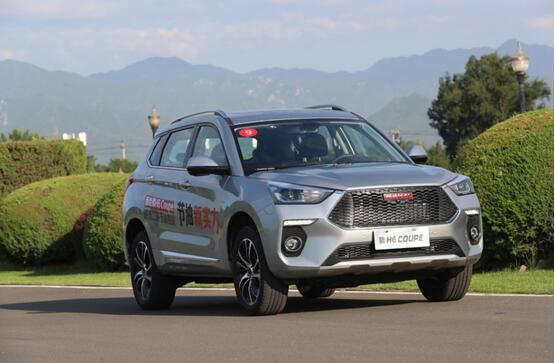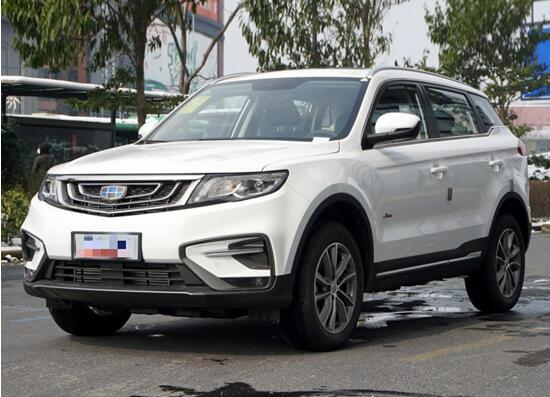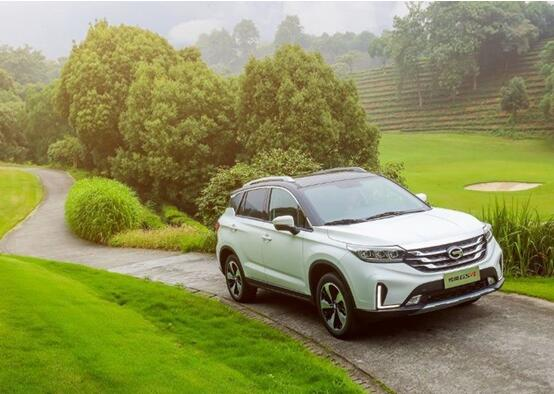在配置方面，我们选择新哈弗H6 Coupe 红标自动两驱超豪型（12.7万）、吉利博越 1.8TD 自动两驱智联型4G互联版（12.98万）和传祺GS4 235T DCT两驱豪华智联百万纪念版（13.38万）这三款价格相近的车型进行比较。

关于实用性配置，三款车型均配备了一键启动+无钥匙进入系统、定速巡航、电子驻车、驾驶席电动座椅调节、四门车窗一键升降（带防夹）等配置，充分保证用车的方便快捷。此外，新哈弗H6 Coupe和传祺GS4均配备了超大尺寸全景天窗，将为驾乘者带来更好的车内视野与驾乘享受，驾车远行更富乐趣；博越则在车联网相对更丰富，这也顺应了当下智联化、车联网的发展趋势。

在动力表现上，三款车有所差别。新哈弗H6 Coupe搭载1.5 GDIT直喷增压发动机，最大功率124kW，最大扭矩为285N•m，相较老款动力进一步提升，油门轻盈灵敏，只需轻踩，动力即可快速传递，而中后段加速，动力更足，表现十分抢眼。7速湿式双离合变速器在平顺性上表现不错，对于抖动和顿挫的控制也很优秀，而且百公里综合工况油耗低至6.8L，燃油经济性非常可观，不过在换挡速度上稍显迟滞，动力总成依然有提升的空间。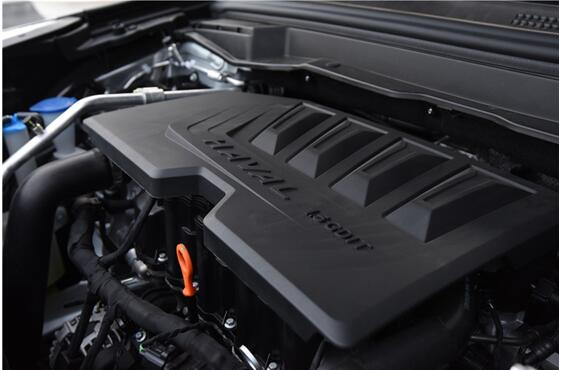2018款吉利博越仍然采用1.8T和2.0L两个排量发动机，其中1.8T发动机有两种调校版本，高功率版本最大功率为135Kw，峰值扭矩285N•m，匹配6速手自一体变速箱，低功率版本最大功率120Kw，峰值扭矩250牛•米，匹配6速手动变速箱。在动力参数上，博越的最大功率略占优势，但新哈弗H6 Coupe以1.5T动力能迸发出与博越1.8T动力同样的最大扭矩，也非常可观。另外，博越在变速箱上同样是倾向平顺性，尽管换挡没有问题，也因此显得不够干脆，较为迟缓，这对于选择了平顺性的车型来说也无可厚非。

传祺GS4依然搭载1.3T和1.5T两款发动机，其中1.5T发动机最大功率为112 kW，最大扭矩为235N•m，在变速箱上，手动挡车型将由5速升级为6速，自动挡车型继续保留爱信6速自动变速箱及7速双离合变速箱。从动力参数上看，传祺GS4都较为逊色，而且在现在追求速度与激情、以年轻人为主的时代，传祺GS4不够强劲的动力表现不足以吸引年轻一代，再加上后期之秀的迎头赶上，传祺GS4依然沿用以往的发动机系统，显有更新换代，恐怕力不从心。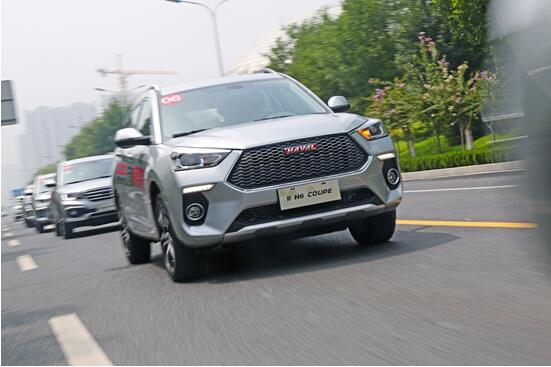通过对外观、空间、配置、动力、安全进行对比，三款车型都实力不俗，但整体而言，新哈弗H6 Coupe较为优秀。尤其是考虑到性价比，虽然三车价位相当，但同等丰富配置下，新哈弗H6 Coupe明显价格更低，性价比更高。外观动感、空间宽裕、配置丰富、动力强劲、安全性高，而且还具备高性价比，作为哈弗H6系列的一员猛将，新哈弗H6 Coupe将以强大实力搅局紧凑型SUV市场。

#### 相关文章

0-500 字已有评论 0条 查看评论>>

### 热门标签

﻿
• 快速找车
• 选择品牌
• 选择品牌
• A  奥迪
• A  阿斯顿·马丁
• A  阿尔法·罗密欧
• B  宝沃
• B  布加迪
• B  巴博斯
• B  保时捷
• B  宾利
• B  奔驰
• B  宝马
• B  本田
• B  别克
• B  标致
• B  比亚迪
• B  宝骏
• B  北汽制造
• B  北汽新能源
• B  北汽幻速
• B  北汽威旺
• B  北京汽车
• B  奔腾
• B  北汽绅宝
• C  长安
• C  长安商用
• C  长城
• C  昌河
• D  大众
• D  道奇
• D  DS
• D  东南
• D  东风风神
• D  东风风行
• D  东风小康
• D  东风风度
• D  东风
• F  福特
• F  丰田
• F  菲亚特
• F  法拉利
• F  福田
• F  福迪
• F  福汽启腾
• G  观致
• G  广汽传祺
• G  广汽吉奥
• G  GMC
• H  红旗
• H  汉腾汽车
• H  哈弗
• H  哈飞
• H  海格
• H  海马
• H  华颂
• H  黄海
• H  华泰
• H  恒天
• J  吉利汽车
• J  捷豹
• J  Jeep
• J  江淮
• J  江铃
• J  金杯
• J  九龙
• J  金旅
• K  凯翼
• K  凯迪拉克
• K  克莱斯勒
• K  科尼塞克
• K  卡威
• K  开瑞
• L  路虎
• L  林肯
• L  劳斯莱斯
• L  兰博基尼
• L  雷克萨斯
• L  铃木
• L  雷诺
• L  理念
• L  力帆
• L  莲花汽车
• L  猎豹
• L  路特斯
• L  陆风
• M  马自达
• M  MG
• M  MINI
• M  玛莎拉蒂
• M  摩根
• M  迈凯轮
• N  纳智捷
• O  欧宝
• O  讴歌
• O  欧朗
• Q  奇瑞
• Q  起亚
• Q  启辰
• R  日产
• R  荣威
• R  瑞麒
• S  三菱
• S  斯威汽车
• S  萨博
• S  smart
• S  斯柯达
• S  斯巴鲁
• S  思铭
• S  双龙
• S  上汽大通
• S  双环
• T  特斯拉
• T  腾势
• W  沃尔沃
• W  五菱汽车
• W  五十铃
• W  威兹曼
• W  威麟
• X  现代
• X  雪佛兰
• X  雪铁龙
• X  西雅特
• Y  一汽
• Y  英菲尼迪
• Y  英致
• Y  依维柯
• Y  野马汽车
• Y  永源
• Z  众泰
• Z  中华
• Z  中兴
• Z  知豆
• 选择车系
• 选择车系
• 车型对比
• 选择品牌
• 选择品牌
• A  奥迪
• A  阿斯顿·马丁
• A  阿尔法·罗密欧
• B  宝沃
• B  布加迪
• B  巴博斯
• B  保时捷
• B  宾利
• B  奔驰
• B  宝马
• B  本田
• B  别克
• B  标致
• B  比亚迪
• B  宝骏
• B  北汽制造
• B  北汽新能源
• B  北汽幻速
• B  北汽威旺
• B  北京汽车
• B  奔腾
• B  北汽绅宝
• C  长安
• C  长安商用
• C  长城
• C  昌河
• D  大众
• D  道奇
• D  DS
• D  东南
• D  东风风神
• D  东风风行
• D  东风小康
• D  东风风度
• D  东风
• F  福特
• F  丰田
• F  菲亚特
• F  法拉利
• F  福田
• F  福迪
• F  福汽启腾
• G  观致
• G  广汽传祺
• G  广汽吉奥
• G  GMC
• H  红旗
• H  汉腾汽车
• H  哈弗
• H  哈飞
• H  海格
• H  海马
• H  华颂
• H  黄海
• H  华泰
• H  恒天
• J  吉利汽车
• J  捷豹
• J  Jeep
• J  江淮
• J  江铃
• J  金杯
• J  九龙
• J  金旅
• K  凯翼
• K  凯迪拉克
• K  克莱斯勒
• K  科尼塞克
• K  卡威
• K  开瑞
• L  路虎
• L  林肯
• L  劳斯莱斯
• L  兰博基尼
• L  雷克萨斯
• L  铃木
• L  雷诺
• L  理念
• L  力帆
• L  莲花汽车
• L  猎豹
• L  路特斯
• L  陆风
• M  马自达
• M  MG
• M  MINI
• M  玛莎拉蒂
• M  摩根
• M  迈凯轮
• N  纳智捷
• O  欧宝
• O  讴歌
• O  欧朗
• Q  奇瑞
• Q  起亚
• Q  启辰
• R  日产
• R  荣威
• R  瑞麒
• S  三菱
• S  斯威汽车
• S  萨博
• S  smart
• S  斯柯达
• S  斯巴鲁
• S  思铭
• S  双龙
• S  上汽大通
• S  双环
• T  特斯拉
• T  腾势
• W  沃尔沃
• W  五菱汽车
• W  五十铃
• W  威兹曼
• W  威麟
• X  现代
• X  雪佛兰
• X  雪铁龙
• X  西雅特
• Y  一汽
• Y  英菲尼迪
• Y  英致
• Y  依维柯
• Y  野马汽车
• Y  永源
• Z  众泰
• Z  中华
• Z  中兴
• Z  知豆
• 选择车系
• 选择车系
• 选择车型
• 选择车型
• 意见反馈